# ISEE Upper Level Math Practice Test 17

### Test Information11 questions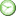0 minutes

Take more free ISEE Mathematics Achievement Practice Tests available from crackssat.com.

1. Steve is measuring the growth of a tomato plant. The chart below indicates his measurements for the past five weeks. Based on the information in the chart, what should he predict will be the height of the plant at week 6?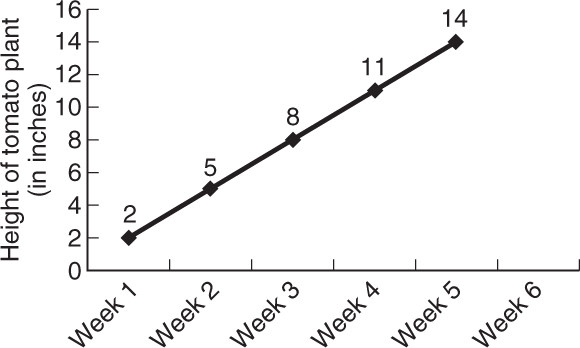• A. 15 inches
• B. 17 inches
• C. 18 inches
• D. 21 inches

2. What digit is in the thousandths place in 5,624.03917?

• A. 5
• B. 3
• C. 9
• D. 1

3. A ladder is placed against a building. If the ladder makes a 55° angle with the ground, what is the measure of the angle that the ladder makes with the building?

• A. 25°
• B. 30°
• C. 35°
• D. 40°

4. If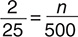, what is n?

• A. 20
• B. 40
• C. 60
• D. 8

5. Each edge of a cube measures 5 in. What is the volume?

• A. 10 in3
• B. 25 in3
• C. 100 in3
• D. 125 in3

6. Renee deposits \$4,000 in a savings account that earns 2% simple interest per year. How much interest will she earn after 2 years?

• A. \$100
• B. \$160
• C. \$165
• D. \$180

7. In the figure shown, the two outer rays form a right angle. If angle 1 measures 65°, what is the measure of angle 2?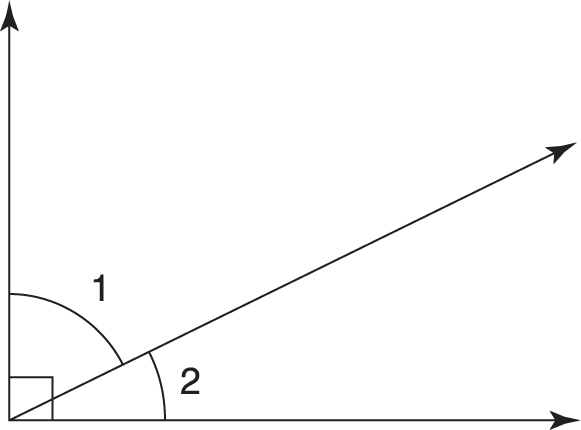• A. 90°
• B. 60°
• C. 35°
• D. 25°

8. Which of the following numbers is evenly divisible by 4?

• A. 8,376,014
• B. 3,027,690
• C. 7,616,550
• D. 5,921,728

9. In the figure shown, if angle 2 measures 45°, what is the measure of angle 4?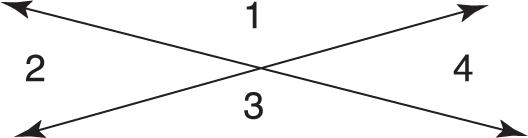• A. 135°
• B. 75°
• C. 45°
• D. 35°

10. Which if the following is divisible only by itself and 1?

• A. 61
• B. 81
• C. 171
• D. 207

11. Figure WXYZ is a parallelogram. Which of the following is NOT necessarily true?• A. Side WX is parallel to side ZY.
• B. Side XY is parallel to side WZ.
• C. ∠W has the same measure as ∠Y.
• D. Side WX is the same length as side XY.